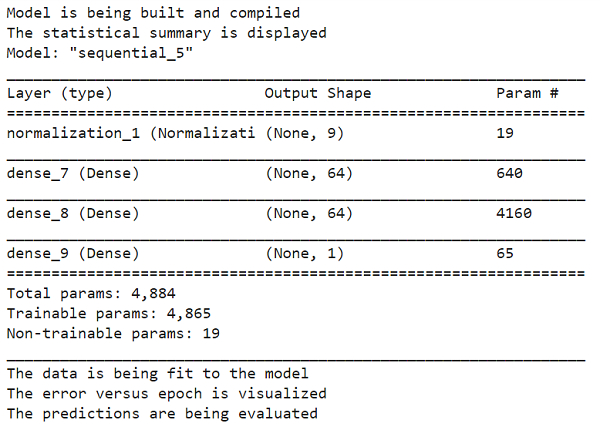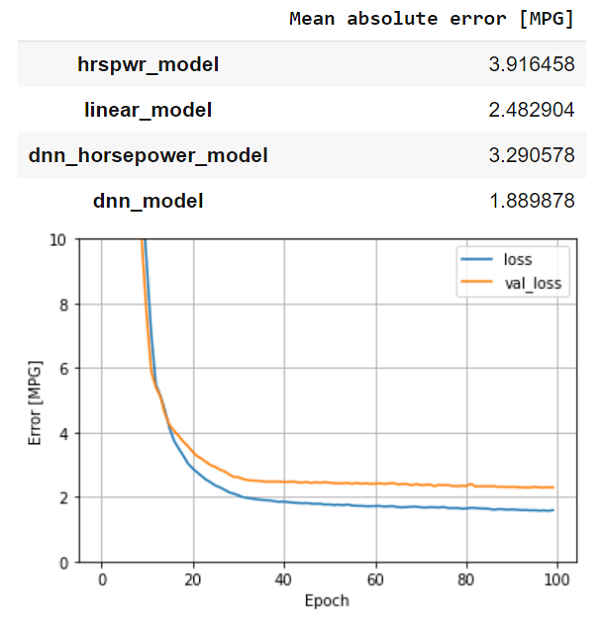# How can a DNN (deep neural network) model be used to predict MPG values on Auto MPG dataset using TensorFlow?

PythonServer Side ProgrammingProgrammingTensorflow

Tensorflow is a machine learning framework that is provided by Google. It is an open−source framework used in conjunction with Python to implement algorithms, deep learning applications and much more. It is used in research and for production purposes. Tensor is a data structure used in TensorFlow. It helps connect edges in a flow diagram. This flow diagram is known as the ‘Data flow graph’. Tensors are nothing but multidimensional array or a list.

The ‘tensorflow’ package can be installed on Windows using the below line of code −

pip install tensorflow

The aim behind a regression problem is to predict the output of a continuous or discrete variable, such as a price, probability, whether it would rain or not and so on.

The dataset we use is called the ‘Auto MPG’ dataset. It contains fuel efficiency of 1970s and 1980s automobiles. It includes attributes like weight, horsepower, displacement, and so on. With this, we need to predict the fuel efficiency of specific vehicles.

We are using the Google Colaboratory to run the below code. Google Colab or Colaboratory helps run Python code over the browser and requires zero configuration and free access to GPUs

(Graphical Processing Units). Colaboratory has been built on top of Jupyter Notebook. Following is the code snippet −

## Example

print("Model is being built and compiled")
dnn_model = build_compile_model(normalizer)
print("The statistical summary is displayed ")
dnn_model.summary()

print("The data is being fit to the model")
history = dnn_model.fit(
train_features, train_labels,
validation_split=0.2,
verbose=0, epochs=100)
print("The error versus epoch is visualized")
plot_loss(history)
print("The predictions are being evaluated")
test_results['dnn_model'] = dnn_model.evaluate(test_features, test_labels, verbose=0)

pd.DataFrame(test_results, index=['Mean absolute error [MPG]']).T

## Output## Explanation

• The model is built and compiled.

• The statistical values like count, mean, median are displayed using the ‘summary’ function.

• This compiled model is fit to the data.

• The visualization of number of steps versus the error in the prediction is plotted on the console.

• Using DNN is better in comparison to linear regression.